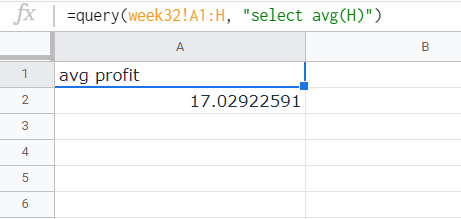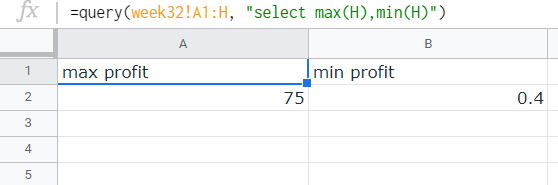# How to Use SQL Query for Aggregate Values in Google Sheets

Learn how to use SQL Queries for aggregate values in Google Sheets to better analyze your data. This tutorial will include how to use SUM, COUNT, AVG, MAX, and MIN.It is possible to query for aggregate values in Google Sheets. Instead of querying entire worksheets and then doing fairly routine calculations, you can shorten the time in calculations and lighten the load in your browser by submitting queries for aggregate values instead. In this tutorial, we will see how the five aggregation functions work in Google Sheets.

## Using SUM() in your query

The SUM() function is used to add the values of a specified range of cells together. If you include it in the query, it will add the cells in a certain column. For example, we have the following sheet that includes the date of transaction, the item bought, the price per item, the amount of items bought, the resulting total price, the cost per item, the total cost, and the profit.Our starting sheet that contains the date of transaction, the item bought, the price per item, the amount of items bought, the resulting total sales, the cost per item, the total cost, and the profit.

We need the total amount of items bought, so we need to apply the SUM() function to the column for the amount of item, which is column D. To do so, we make the following query (without quotes):

"select sum(D)"

With this, we'll have to place it in Google Sheets' QUERY function with the format:

=query([range],"[SQL query]'")

The result will look like this:

Since there is only a single sum for each column, the result is essentially a single-cell sheet.

## Using COUNT() in your query

The COUNT() function counts all the rows with entries in the specified column, skipping the rows that are blank. The query calling for the total number of entries in a column would look similar to that of the SUM() function (without quotes):

"select count(D)"

With this, we'll have to place it in Google Sheets' QUERY function with the format:

=query([range],"[SQL query]'")

The result will look like this:

## Using AVG() in your query

The AVG() function will calculate the average value for the entire column. As an example, we want to calculate the average profit per order. To do so, we make the following query (without quotes):

"select avg(H)"

With this, we'll have to place it in Google Sheets' QUERY function with the format:

=query([range],"[SQL query]'")

The result will look like this:Query result for the average profit per order. Note that the value is not yet formatted to reflect the value as currency.

## Using MAX() and MIN() in your query

The MAX() and MIN() functions look for and display the maximum and minimum value in the selected column, respectively. As an example, we wish to find the maximum and minimum profit that we got from a single order in the period specified in the entire sheet. To do so, we make the following query (without quotes):

"select max(H), min(H)"

With this, we'll have to place it in Google Sheets' QUERY function with the format:

=query([range],"[SQL query]'")

The result will look like this:Query result for the maximum and minimum profit from a single order. Note that the values are not formatted to reflect the value as currency.

It also works with dates:

## How about calculating, let’s say, the sum per day?

We can use these aggregate functions alongside the group by clause in our query. Head to this tutorial to see how the puzzle pieces fit!

## Too much of a hassle?

While this lets us analyze large chunks of data much easier, it will take us a lot of time to do so. Maybe there is a better way so that all the analysis is automated and we will just have to make important decisions for our business…

If that’s what you fancy, consider trying Lido. With a few clicks, you can now access all the relevant metrics without going through the hassle of accessing the SQL databases of your eCommerce platforms and then coding the formulas to process them. Let our platform do it all for you!